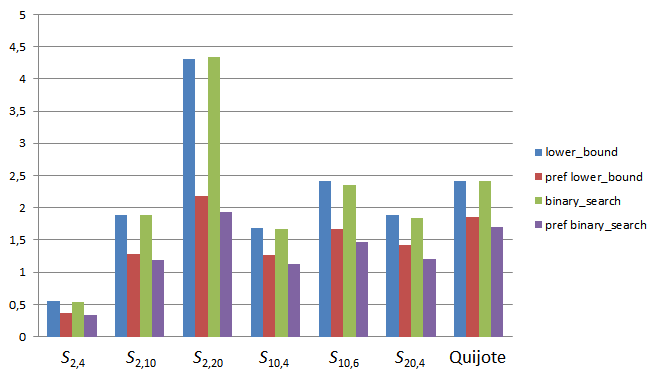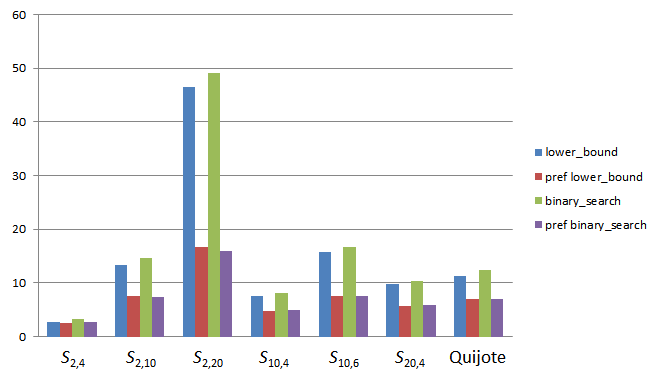## Saturday, April 12, 2014

### Climbing down the tree: framework and performance

Continuing with our study on optimizing lexicographical comparison as used within binary search algorithms, we will provide now a fuller description of the supporting C++ framework and measure the actual performance with several test sequences on different environments.
Let Compare be a binary relation inducing a strict weak ordering on values of a type T, cmp a value of Compare and x a value of T. A binary search binder for cmp and x is any object cbind for which the expressions cbind.less(y) and cbind.greater(y) are valid whenever cmp(y,x) and cmp(x,y) are valid, respectively, and return the same boolean value as these. Moreover, when the range being searched is sorted (i.e. not merely partitioned with respect to x) the implementation of a binary search binder can assume the following usage restrictions:
• If either the invocation cbind.less(y) returns true or the invocation cbind.greater(y) returns false, subsequent invocations on cbind will be done against values not less than y under the ordering relation induced by Compare.
• If either the invocation cbind.less(y) returns false or the invocation cbind.greater(y) returns true, subsequent invocations on cbind will be done against values not greater than y.
Standard C++ binary search algorithms such as lower_bound or binary_search can be implemented by replacing each usage of the given Compare object with the equivalent invocation of a single associated binder obtained through comp_bind, which is defined as:
```template<typename Comp,typename T>
struct comp_binder
{
comp_binder(Comp cmp,const T& x):cmp(cmp),x(x){}

template<typename Q> bool less(const Q& y){return cmp(y,x);}
template<typename Q> bool greater(const Q& y){return cmp(x,y);}

Comp     cmp;
const T& x;
};

template<typename Comp,typename T>
comp_binder<Comp,T> comp_bind(Comp cmp,const T& x)
{
return comp_binder<Comp,T>(cmp,x);
}
```
(Note that comp_binder is compatible even with polymorphic comparison types such as C++14 transparent operator functors.) While providing backwards compatibility, this framework introduces new customization opportunities via specialization of comp_binder; this is precisely what prefix_less does:
```template<typename T>
struct prefix_less:std::less<T>
{
};

template<typename T>
struct comp_binder<prefix_less<T>,T>
{
bool less(const T& y);
bool greater(const T& y);
};
```
T must be either an instantiation of std::basic_string or a container with lexicographical less-than semantics. prefix_less<T> is functionally equivalent to std::less<T>, but its associated binder takes advantage of the special binary search context where it is being used to provide better algorithmic complexity. The actual implementation can be consulted in the test program provided below. From the point of view of the end user, prefix_less works exactly as std::less:
```std::vector<std::string> v;
...
auto it=std::lower_bound(
v.begin(),v.end(),x,prefix_less<std::string>());
```
except that, if the algorithm internally uses comp_bind, the resulting performance might improve —or degrade, we will see now how the real thing behaves.
Measurements
I have written a program that compares the execution speeds of std::less and prefix_less with comp_bind-compliant versions of lower_bound and binary_search under the following testing scenarios:
```template<typename Sequence,typename Compare>
void run_lower_bound(const Sequence& s,Compare cmp)
{
for(const auto& x:s){
lower_bound(s.begin(),s.end(),x,cmp);
}
}

template<typename Sequence,typename Compare>
void run_binary_search(const Sequence& s,Compare cmp)
{
for(const auto& x:s){
binary_search(s.begin(),s.end(),x,cmp);
}
}
```
An assortment of sequences is tested (in what follows SN,L denotes the sorted range of strings of length L composed with the N characters '0', '1', ...; for instance, S2,3 is the range ["000", "001", "010", "011", "100", "101", "110", "111"]):
• S2,4 (size: 16), S2,10 (size: 1,024), S2,20 (size: ~1,000,000), S10,4 (size: 10,000), S10,6 (size: 10,000,000), S20,4 (size: 160,000),
• The sorted set of ~23,000 unique words within Cervantes' Don Quijote de la Mancha
Several representation types are also used to encode the elements of these sequences:
• std::string,
• std::wstring,
• std::vector<udchar>, where udchar is a simple wrapper around char,
• std::vector<std::string>, i.e. each character is codified as a separate string of length 1.
MSVC
Tests were built with Microsoft Visual Studio 2012 using default release mode settings and run on a Windows box with an Intel Core i5-2520M CPU @2.50GHz. Depicted values are microseconds/n, where n is the number of elements in the sequence tested.Execution times, std::string.Execution times, std::wstring.Execution times, std::vector.Execution times, std::vector.
It is hard to beat the default implementation of lexicographical comparison for such simple types as std::string, which usually reduces to a call to the extremely efficient std::memcmp, and additionally the theoretical performance gain of prefix_less is dwarfed by the constant bookkeeping cost associated to tracking the length of the discarded common prefix. With these considerations in mind, prefix_less performs only marginally better than std::less when average string length is small and the representation type is simple, and excels in the opposite situations. std::vector<udchar> is an anomaly for which I do not have a clear explanation: udchar not being a memcmp-compatible type, one would expect prefix_less to behave unconditionally better than std::less just as with std::vector<std::string>, but in fact this is not the case; maybe there is some inlining threshold met by prefix_less that the simpler code of std::less manages to avoid.
GCC
Thomas Goyne has built the tests with GCC 4.9 20140330, release settings -O3 -std=c++11, and run them on an OS X 10.9.1 box with an Intel Core i7-3720QM @2.6GHz.Execution times, std::string.Execution times, std::wstring.Execution times, std::vector.Execution times, std::vector.
Unlike with MSVC, here prefix_less performs consistently better than std::less except for S2,4, where string length is too small to compensate for the extra constant cost incurred by prefix_less. Reductions in execution times range from 10% to 50%.
Clang
Results also provided by Thomas Goyne: Clang 503.0.38 (3.4svn) with libc++, release settings -O3 -std=c++11, same OS X machine as with GCC.Execution times, std::string.Execution times, std::wstring.Execution times, std::vector.Execution times, std::vector.
Performance gains are similar to those obtained in the case of GCC except when the representation type is std::vector<udchar>, in which case std::less is generally faster, again for reasons for which I do not have a satisfactory explanation.
Conclusions
C++ binary search algorithms can be extended in a backwards compatible way to support mechanisms for lexicographical comparison with lower complexity and, generally speaking, better performance than provided by regular std::less instantiations. Although we have focused on comparison of containers and strings, there is also an additional category of entities, namely tuples and similar types, that could also benefit from this framework: this we might explore in future entries.